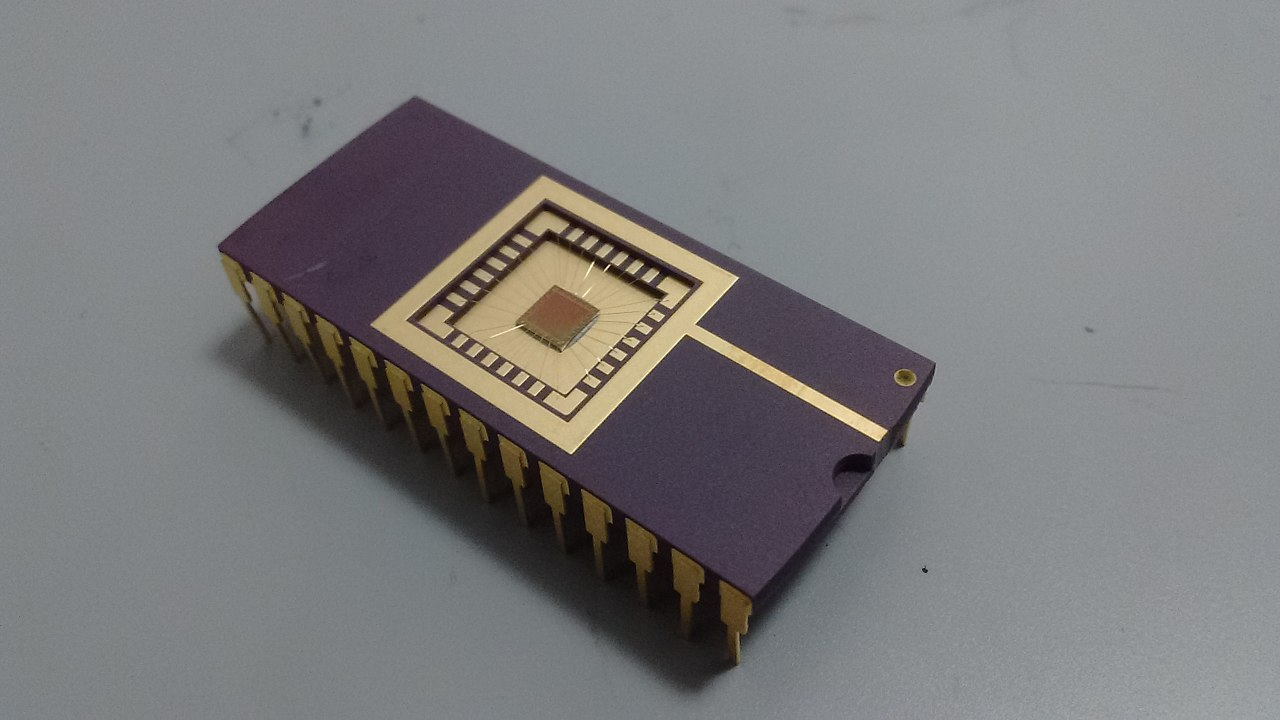×

Search anything:

# One Instruction Set Computer (OISC)

#### instruction set oisc Computer ArchitectureGet this book -> Problems on Array: For Interviews and Competitive Programming

As the name suggest, this category of computing devices has only one instruction in its instruction set. At a first glance, this may seem impossible and you may think over how a minimum of two distinct symbols are needed to represent an information (in connection to binary numbers).

This might seem to be revolutionary and it is easy to understand at the same time. It is based on some simple principles which we will take a look at.

One instruction set computer (OISC) (also known as ultimate reduced instruction set computer (URISC)) is a computer architecture based on two fundamental ideas:

• One instruction in instruction set
• memory-mapping registers
• self-modifying code

There are three approaches to implementing OISC:

• Bit-manipulating machines
• Transport triggered architecture machines
• Arithmetic-based Turing-complete machines

Cryptoleq processor based on OISC concepts:### Explanation

A typical program in an assembly language consists of code which operates on data. The code is normally made up of a sequence of commands/instructions, and typically, most of those commands will take arguments.

The OISC (One Instruction Set Computer) concept maintains this construction. However, it only has one sort of instruction/command, which is therefore entirely defined by its arguments. Most OISC commands are moderately, but not excessively, complex and take many arguments. OISCs also commonly use techniques such as self-modifying code and memory-mapping important registers, in order to gain more functionality from their instruction.

### Instruction types

One of the questions at this stage will be: What kind of single instruction is used in OSIC?

Following are common choices of instructions:

• Subtract and branch if less than or equal to zero
• Subtract and branch if negative
• Subtract if positive else branch
• Reverse subtract and skip if borrow
• Move (used as part of a transport triggered architecture)
• Subtract and branch if non zero (SBNZ a, b, c, destination)
• Cryptoleq (heterogeneous encrypted and unencrypted computation)

Note that only one of the above instruction is used in a particular implementation or version of OSIC.

Consider this example with only one instruction:

``````
subleq: Subtract and branch if less than or equal to zero
subleq a, b, c   ; Mem[b] = Mem[b] - Mem[a]
; if (Mem[b] ≤ 0) goto c
``````

Using the above example, we will demonstrate how we can implement high level instructions such as:

• JUMP
• MOVE
• NOT

JUMP

We would like to have an alternative to command JMP a which jumps to memory address a.

``````
subleq Z, Z, a
``````

We would implement the command ADD a, b which does binary add on data at a and b

``````
subleq a, Z
subleq Z, b
subleq Z, Z
``````

MOVE

We would implement the command MOV a, b which will move contents at a to b

``````
subleq b, b
subleq a, Z
subleq Z, b
subleq Z, Z
``````

NOT

We would implement the binary not operation

``````
subleq2 tmp          ; tmp = 0 (tmp = temporary register)
subleq2 tmp
subleq2 minus_one    ; acc = -1
subleq2 a            ; a' = a + 1
subleq2 Z            ; Z = - a - 1
subleq2 tmp          ; tmp = a + 1
subleq2 a            ; a' = 0
subleq2 tmp          ; load tmp into acc
subleq2 a            ; a' = - a - 1 ( = ~a )
subleq2 Z            ; set Z back to 0
``````

### Commercial Use

OISC is used as:

• There is a compiler called Higher Subleq written by Oleg Mazonka that compiles a simplified C program into subleq code.
• Cryptoleq is an OISC language

OISC has several advantages such as:

• There is no need for a machine language opcode
• OISC is capable of being a universal computer in the same manner as traditional computers that have multiple instructions
• Used in teaching computer architecture
• Used as computational models in structural computing research#### OpenGenus Foundation

The official account of OpenGenus IQ backed by GitHub, DigitalOcean and Discourse

Improved & Reviewed by:

One Instruction Set Computer (OISC)